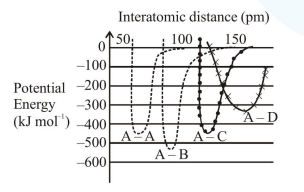# The intermolecular potential energy for the molecules A, B, C

Question:

The intermolecular potential energy for the molecules A, B, C and D given below suggests that :1. $\mathrm{D}$ is more electronegative than other atoms

2. A-D has the shortest bond length

3. A-B has the stiffest bone

4.  $\mathrm{A}-\mathrm{A}$ has the largest bond enthalpy

Correct Option: , 3

Solution:

From the given graph, potential energy of A-B molecule is minimum.

Thus A-B bond is most stable and have strongest bond amongst these.

$\mathrm{B} \rightarrow$ Most electronegative

$\mathrm{D} \rightarrow$ Least electronegative

$\mathrm{A}-\mathrm{B} \rightarrow$ Shortest bond length

A-B $\rightarrow$ Largest bond enthalpy

Therefore correct option is (3).